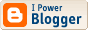## A Challenge to Rothbardians Everywhere

Oh boy, a contest!  Current events are too depressing these days.  Let's shift gears for a bit and move to more enjoyable matters, like one of our occasional contests (which are, admittedly, strained efforts to see if we actually have any readers).  I just happened to notice today that someone ("purplearcanist") commented on an old and infamous post of mine, one of the ones in which I critiqued some of the economics promoted by Murray Rothbard and his LvMI acolytes.  I've gone ahead and responded, probably a few years late, to purpleacrcanist's comments; I appreciate that s/he thought it sufficiently important to respond, even if I do find the responses weak at best.

One of the points purplearcanist raises is the objection that the neoclassical conditions for utility maximization are hopelessly confused because of the "incommensurate units" problem. Marginal utilities and prices are measured in different units, so MUX/MUY = PX/Pis incoherent.  I dispense with this confusion (for the umpteenth time, and I'm hardly the first.)  But this reminds me of an amusing puzzle that's vaguely related to the "incommensurate units" problem.

Suppose the following.  In your left pocket you have 10 cents.  In your right pocket you have 1/10 of a dollar.  Square each amount.  Now how much do you have in each pocket?

Readers are invited to post their answers in the comments section.  There are two categories in which answers can compete.  1. The best answer using Hulsmann's theory of units (see pp. 9-10, "Cardinality") to circumvent the apparent anomaly in the problem wins.  "Best" will be the one I find most consistent, creative, and entertaining.  My decision will be unassailable and final. 2. The first correct answer posted also wins.  I suppose that, as in the past, we'll award some sort of prizes to winners.  (Yes, real prizes actually have been awarded!)

Contest deadline: 29 July 2012, 22:00 MDT.

I suspect you'll have to be a true believer in Rothbard/Hulsmann theory to pull off a win in category 1.

Update: 1. I am heading into the mountains for a week; when I return I will judge entries, if any.  2. Someone asked me if the contest is open to anyone. Yes.

This comment has been removed by the author.

Here is my feeble attmept to answer these questions. Incorporating Hulsmann's theory was difficult, mainly because I don't understand his critique very well.

c = cents
\$ = dollars
^2 = squared

(10c)^2 = 100c^2

(1/10\$)^2 = 1/100\$^2

Therefore, you have 100 cents squared in your left pocket, and 1/100 of a dollar squared in your right pocket.

The correct answer to how much money is in your pocket after multiplying 10 cents by 10 cents and 1/10 of a dollar by 1/10 of a dollar is nothing, since "cents squared" and "dollars squared" are nonsensical units. There is no unit of currency that conforms to squared dimensions.

If the question were to multiply by 10 each amount, then the answers would be 100 cents, and 1 dollar.

On the other ahdn, if instead of cents and dollars the amounts were simply 1/10 and .1 (10 percent), then the answers would be 1/00 and .01.

In the above two examples, the results remain equal. But you can square neither a cent nor a dollar. You can mutliply cents and dollars by numbers, but you cannot multiply them by themselves.

The conclusion I am left with is that the question itself is a nonstarter, because squaring nondimensional units is impossible. If I am wrong Dr. Steele, I'll give you 10,000 dollars squared.

Left pocket: (10c)^2 = 100 c^2
Right pocket: [(1/10)\$]^2 = (1/100)\$^2

If we let 1\$=100c, then you have (1/100)*100c*100c = 100c^2

So, in both pockets, you have 100c^2.

But, of course this makes no sense (cents? ha.) Dollars and cents are one dimensional units and we have no concept of what square dollars or square cents are (we don't know what they look like in two dimensions).

Whatever it is (we don't have a real concept), you have the same amount in each pocket, since you can give them the same units from the beginning. 1/10 of a dollar is 10 cents.